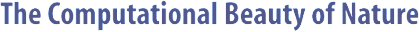Computer Explorations of Fractals, Chaos,Complex Systems, and Adaptation· title page · home* · cover artwork · jacket text · table of contents · the author* · ordering informationBook Contents
 · three themes · part synopses · selected excerpts · all figures from book · quotes from book · glossary from book · bibliography · slide showSource Code
 · overview &documentation · FAQ list* · download source code · java appletsMiscellany
 · news* · reviews & awards · errata · for educators · bibliography (BibTeX format) · other linksMG Documentation

```

```

#### NAME

```       mg - plot the phase space of the Mackey-Glass system

```

#### SYNOPSIS

```       mg -help
or
mg     [-width  integer] [-height integer] [-skip integer]
[-points integer] [-delta integer]  [-tau  integer]
[-A  double]  [-B double] [-dt double] [-x0 double]
[-factor  double]  [-data]  [-inv]  [-mag  integer]
[-term string]

```

#### DESCRIPTION

```       The  phase  space  of  the  Mackey-Glass  system, which is
described by the delay differential equation

dx(t)/dt = A * x(t-Tau) / (1 + x(t-Tau)^10) - B * x(t),

is plotted according to the specified parameters.  The  x-
coordinate  of the plot is determined by x(t) while the y-
coordinate is determined by x(t-delta).

```

#### OPTIONS

```       -width integer
Width of the plot in pixels.

-height integer
Height of the plot in pixels.

-skip integer
Number of initial points to skip.

-points integer
Number of points to plot.

-delta integer
Time steps to delay for.

-tau integer
Value of the Tau parameter.

-A double
Value of the A parameter.

-B double
Value of the B parameter.

-dt double
Time step size.

-x0 double
Initial X value.

-factor double
Auto-scale expansion factor.

-data  Don't plot, but print points.

-inv   Invert all colors?

-mag integer
Magnification factor.

-term string
How to plot points.

```

#### MISCELLANY

```       The plot region is determined by the points that are  ini-
tially  skipped.  If this number is too small (i.e., it is
not very representative of the range of the  plotted  val-
ues),  then  you may need to increase the number specified
by the -skip option.  Alternatively, you  can  adjust  the
value   given   to   -factor,  which  simply  fractionally
increases the border of the plot.

The program uses a second-order Euler's method to  perform
the  numerical integration, which is sufficient for simple

```

#### BUGS

```       No sanity checks are performed to make sure  that  any  of
the options make sense.

```

#### AUTHOR

```       Copyright (c) 1997, Gary William Flake.

Permission  granted  for any use according to the standard
GNU ``copyleft'' agreement provided that the author's com-
ments  are  neither  modified nor removed.  No warranty is
given or implied.

```Copyright © Gary William Flake, 1998-2002. All Rights Reserved.Last modified: 30 Nov 2002